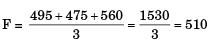Courses

# Past Year Questions: Forecasting

## 20 Questions MCQ Test Engineering Mechanics | Past Year Questions: Forecasting

Description
This mock test of Past Year Questions: Forecasting for Mechanical Engineering helps you for every Mechanical Engineering entrance exam. This contains 20 Multiple Choice Questions for Mechanical Engineering Past Year Questions: Forecasting (mcq) to study with solutions a complete question bank. The solved questions answers in this Past Year Questions: Forecasting quiz give you a good mix of easy questions and tough questions. Mechanical Engineering students definitely take this Past Year Questions: Forecasting exercise for a better result in the exam. You can find other Past Year Questions: Forecasting extra questions, long questions & short questions for Mechanical Engineering on EduRev as well by searching above.
QUESTION: 1

Solution:
QUESTION: 2

Solution:
QUESTION: 3

### In a forecasting model, at the end of period 13, the forecasted value for period 14 is 75. Actual value in the periods 14 to 16 are constant at 100. If the assumed simple exponential smoothing parameter is 0.5, then the MSE at the end of period 16 is 

Solution:

F14 = 75, D14 = 100
F15 = F14 + a (D14 - F14)
= 75 + 0.5 (100.75)
F15 = 87.5
D15 = 100
F16 = 87.5 + 0.5 (100 – 87.5)
F16 = 93.75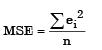Where ei = Di – Fi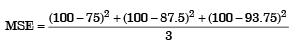MSE = 273.44

QUESTION: 4

The most commonly used criteria for measuring forecast error is



Solution:
QUESTION: 5

In a time series forecasting model, the demand for five time periods was 10,13,15,18 and 22. A linear regression fit resulted in an equation F =  6.9 + 2.9 where F is the forecast for period f.
The sum of absolute deviations for the five data is



Solution:

F = 6.9 + 2.9 t
F1 = 6.9 + 2.9 × 1 ⇒ 9.8
F2 = 6.9 + 2.9 × 2 ⇒ 12.7
F3 = 6.9 + 2.9 × 3 ⇒ 15.6
F4 = 6.9 + 2.9 × 4 ⇒ 18.5
F5 = 6.9 + 2.9 × 5 = 21.4
D1 = 10 D2 = 13 03 = 15
D4 = 16 D5 = 22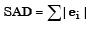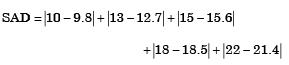Absolute Deviation = 2.2

QUESTION: 6

When using a simple moving average to forecast demand, one would



Solution:
QUESTION: 7

A regression model is used to express a variable V as a function of another variable X, this implies that



Solution:
QUESTION: 8

The sale of cycles in a shop in four consecutive months are given as 70, 68, 82, 95.
Exponentially smoothing average method with a smoothing factor of 0.4 is used in forecasting.
The expected number of sales in the next month is



Solution: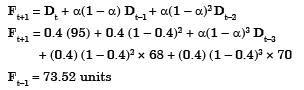QUESTION: 9

For a product, the forecast and the actual sales for December 2002 were 25 and 20 respectively.
If the exponential smoothing constant (a) is taken as 0.2, the forecast sales for January 2003 would be



Solution: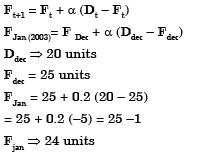QUESTION: 10

The sales of a product during the last four years were 860, 880,870 and 890 units. The forecast for the fourth year was 876 units. If the forecast for the fifth year, using simple exponential smoothing, is equal to the forecast using a three period moving average the value of the exponential smoothing constant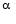is



Solution:

F 4 = 876 units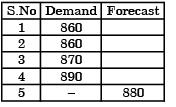F5 = F3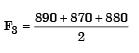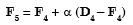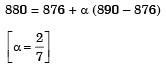QUESTION: 11

In an MRP system, component demand is



Solution:
QUESTION: 12

A moving average system is used for forecasting weekly demand. F1(t) and F2(t) are sequences of forecasts with parameters m1 and m2, respectively, where m1 and m2 (m1 > m2)  denote the numbers of weeks over which the moving averages are taken. The actual demand shows a step increase



Solution:

Gi ven that , at certain the demand increased from d1 to d2 The weightage of the latest demand should be thereface f2(t) become M2 > M2.

QUESTION: 13

Which of the following forecasting methods takes a fraction of forecast error into account for the next period forecast?



Solution:
QUESTION: 14

The demand and forecast for February are 12000 and 10275, respectively. Using single exponential smoothening method (smoothening coefficient = 0.25), forecast for the month of March is



Solution: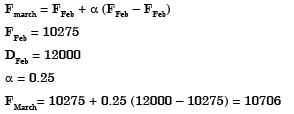QUESTION: 15

In simple exponential smoothing forecasting, to give higher weightage to recent demand information, the smoothing constant must be close to



Solution:

We know that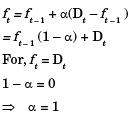Conclusion:
It is showing the limit of responsiveness

QUESTION: 16

In exponential smoothening method, which one of the following is true?



Solution: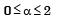high value of ‘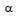means more weightage for immediate forecast.
Less value of ‘means relatively less weightage for immediate forecast, or almost equal weightage for all previous forecast.
Hence high value of forecast is only chosen when nature of demand is not reliable rather unstable.

QUESTION: 17

Sales data of a product is given in the following table: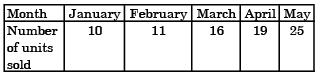Regarding forecast for the month of June, which one of the following statements is TRUE?



Solution:
QUESTION: 18

The demand for a two-wheeler was 900 units and 1030 units in April 2015 and May 2015, respectively.
The forecast for the month of April 2015 was 850 units. Considering a smoothing constant of 0.6, the forecast for the month of June 2015 is



Solution: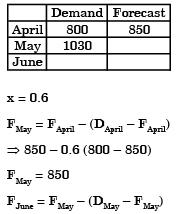= 850 – 0.6 (1030 – 850)
⇒ 850 - 90
⇒ 970 units

QUESTION: 19

The time series forecasting method that gives equal weightage to each of the most recent observation is



Solution:

(a) Simple moving average method gi ves equal weight to each of the most recent observation
(b) Weighted moving average method gi ves more weight to the recent values.
(c) Triple exponential smoothing is a rul e of thumb technique for smoothing time series data using the exponential window function.
(d) Kalman filter is also used for time ser ies data using adaptive models.

QUESTION: 20

The table presents the demand of a product .By simple three-months moving aver age method, the demand-forecast of the product for the month of September is



Solution:

Three month moving average.
Forecast of the product for th e month of September is given by,